SSC BOARD PAPERS IMPORTANT TOPICS COVERED FOR BOARD EXAM 2024

### Linear Equations In Two Variables Class 9th Mathematics Part I MHB Solution

##### Linear Equations In Two Variables Class 9th Mathematics Part I MHB Solution

###### Practice Set 5.1
Question 1.

By using variables x and y form any five linear equations in two variables.

a) x + y = 5

b) x + 2y = 6

c) 2x + y = 4

d) 3x + 4y = 8

e) 5x + 9y = 1

Question 2.

Write five solutions of the equation x + y = 7.

(a)

Let x = 1,

As, x + y = 7

⇒ 1 + y = 7

⇒ y = 6

Hence, solution is x = 1 and y = 6.

(b)

Let x = 2,

As, x + y = 7

⇒ 2 + y = 7

⇒ y = 5

Hence, solution is x = 2 and y = 5.

(c)

Let x = 3,

As, x + y = 7

⇒ 3 + y = 7

⇒ y = 4

Hence, solution is x = 3 and y = 4.

(d)

Let x = 4,

As, x + y = 7

⇒ 4 + y = 7

⇒ y = 3

Hence, solution is x = 4 and y = 3.

(e)

Let x = 5,

As, x + y = 7

⇒ 5 + y = 7

⇒ y = 2

Hence, solution is x = 5 and y = 2.

Question 3.

Solve the following sets of simultaneous equations.

i. x + y = 4; 2x - 5y = 1

ii. 2x + y =5; 3x – y =5

iii. 3x – 5y =16; x - 3y = 8

iv. 2y – x =0; 10x + 15y =105

v. 2x +3y + 4 = 0; x – 5y = 11

vi. 2x – 7y =7; 3x + y =22

(i)

x + y = 4 eq.

2x - 5y = 1 eq.

We can write eq. as,

x = 4 - y eq.

Substituting eq. in eq.,

⇒ 2(4 - y) - 5y = 1

⇒ 8 - 2y - 5y = 1

⇒ -7y = -7

⇒ y = 1

Substituting 'y' in eq.

⇒ x = 4 - 1

⇒ x = 3

Hence, solution is x = 3 and y = 1.

(ii)

2x + y = 5 eq.

3x - y = 5 eq.

We can write eq. as,

y = 5 - 2x eq.

Substituting eq. in eq.,

⇒ 3x - (5 - 2x) = 5

⇒ 3x - 5 + 2x = 5

⇒ 5x = 10

⇒ x = 2

Substituting 'x' in eq.

⇒ y = 5 - 2(2)

⇒ y = 1

Hence, solution is x = 2 and y = 1.

(iii)

3x - 5y = 16 eq.

x - 3y = 8 eq.

We can write eq. as,

x = 8 + 3y eq.

Substituting eq. in eq.,

⇒ 3(8 + 3y) - 5y = 16

⇒ 24 + 9y - 5y = 16

⇒ 4y = -8

⇒ y = -2

Substituting 'y' in eq.

⇒ x = 8 + 3(-2)

⇒ x = 8 - 6 = 2

Hence, solution is x = 2 and y = -2

(iv)

2y - x = 0 eq.

10x + 15y = 105 eq.

We can write eq. as,

x = 2y eq.

Substituting eq. in eq.,

⇒ 10(2y) + 15y = 105

⇒ 20y + 15y = 105

⇒ 35y = 105

⇒ y = 3

Substituting 'y' in eq.

⇒ x = 2(3)

⇒ x = 6

Hence, solution is x = 6 and y = 3.

(v)

2x + 3y + 4 = 0 eq.

x - 5y = 11 eq.

We can write eq. as,

x = 11 + 5y eq.

Substituting eq. in eq.,

⇒ 2(11 + 5y) + 3y + 4 = 0

⇒ 22 + 10y + 3y + 4 = 0

⇒ 13y + 26 = 0

⇒ 13y = -26

⇒ y = -2

Substituting 'y' in eq.

⇒ x = 11 + 5(-2)

⇒ x = 11 - 10 = 1

Hence, solution is x = 1 and y = -2.

(vi)

2x - 7y = 7 eq.

3x + y = 22 eq.

We can write eq. as,

y = 22 - 3x eq.

Substituting eq. in eq.,

⇒ 2x - 7(22- 3x) = 7

⇒ 2x - 154 + 21x = 7

⇒ 23x = 161

⇒ x = 7

Substituting 'x' in eq.

⇒ y = 22 - 3(7)

⇒ y = 22 - 21 = 1

Hence, solution is x = 7 and y = 1.

###### Practice Set 5.2
Question 1.

In an envelope there are some 5 rupee notes and some 10 rupee notes. Total amount of these notes together is 350 rupees. Number of 5 rupee notes are less by 10 than number of 10 rupee notes. Then find the number of 5 rupee and 10 rupee notes.

Let the number of 5 rupees notes = x

Let the number of 10 rupees notes = y

Given, Total amount is 350 Rupees

⇒ 5x + 10y = 350 eq.

Also,

Number of 5 rupees notes are less by 10 than number of 10 rupees note,

y = x - 10

⇒ x = y + 10 eq.

Putting  in 

⇒ 5(y + 10) + 10y = 350

⇒ 5y + 50 + 10y = 350

⇒ 15y = 300

⇒ y = 20

Then, x = y + 10

⇒ x = 20 + 10

⇒ x = 30.

Answer: 30 notes of Rs 5 and 20 notes of Rs. 10.

Question 2.

The denominator of a fraction is 1 more than twice its numerator. If 1 is added to numerator and denominator respectively, the ratio of numerator to denominator is
1 : 2. Find the fraction.

Let the numerator be 'x' and denominator be 'y'

Given,

The denominator of a fraction is 1 more than twice its numerator

⇒ y =2 x + 1

⇒ y - 2 x = 1 ....... (1)

If 1 is added to numerator and denomination, the ratio of the numerator to denominator becomes 1:2.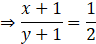⇒ 2(x + 1) = y + 1

⇒ 2x + 2 = y + 1

⇒ y - 2x = 1 ....... (2)

As (1) and (2) are the same, there can be infinitely many solutions for x and y.

One such solution is:

x = 4 and y = 9

Now,

y - 2 x = 9 - 2(4)
= 9 - 8
= 1

Question 3.

The sum of ages of Priyanka and Deepika is 34 years. Priyanka is elder to Deepika by 6 years. Then find their today's ages.

Let the ages of Priyanka and Deepika be 'x' and 'y' respectively.

Given,

Sum of ages is 34

⇒ x + y = 34

⇒ y = 34 - x eq.

Also, Priyanka is elder to Deepika by 6 years

⇒ x = y + 6

Using eq. we have

⇒ x = 34 - x + 6

⇒ 2x = 40

⇒ x = 20

Putting this value in eq.

⇒ y = 34 - 20 = 14 years.

Hence, Age of Priyanka = x = 20 Years

Age of Deepika = y = 14 years.

Question 4.

The total number of lions and peacocks in a certain zoo is 50. The total number of their legs is 140. Then find the number of lions and peacocks in the zoo.

Let the number of lions be 'x' and peacocks be 'y'

Given, Total no of lions and peacocks is 50

⇒ x + y = 50

⇒ x = 50 - y eq.

Also, Total no of their legs is 140, as lion has four legs and peacocks has 2 legs

⇒ 4x + 2y = 140

⇒ 4(50 - y) + 2y = 140

⇒ 200 - 4y + 2y = 140

⇒ 2y = 60

⇒ y = 30

Using this in eq.

⇒ x = 50 - 30 = 20

Therefore,

No of lions, x = 20

No of peacocks, y = 30

Question 5.

Sanjay gets fixed monthly income. Every year there is a certain increment in his salary. After 4 years, his monthly salary was Rs. 4500 and after 10 years his monthly salary became 5400 rupees, then find his original salary and yearly increment.

Let the original salary be 'x' and yearly increment be 'y'

After 4 years, his salary was Rs. 4500

⇒ x + 4y = 4500

⇒ x = 4500 - 4y eq.

After 10 years, his salary becomes 5400

⇒ x + 10y = 5400

⇒ 4500 - 4y + 10y = 5400

⇒ 6y = 900

⇒ y = 150

Putting this in eq.,

⇒ x = 4500 - 4(150)

⇒ x = 4500 - 600 = 3900

Hence, his original salary was Rs. 3900 and increment per year was 150 Rs.

Question 6.

The price of 3 chairs and 2 tables is 4500 rupees and price of 5 chairs and 3 tables is 7000 rupees, then find the price of 2 chairs and 2 tables.

Let the price of one chair be 'x' and one table be 'y'.

Given,

Price of 3 chairs and 2 tables = 4500 Rs

⇒ 3x + 2y = 4500

Multiplying by 3 both side,

⇒ 9x + 6y = 13500

⇒ 6y = 13500 - 9x eq.

Price of 5 chairs and 3 tables = 7000 Rs

⇒ 5x + 3y = 7000

Multiplying by eq. both side,

⇒ 10x + 6y = 14000

⇒ 10x + 13500 - 9x = 14000 eq.[From 1]

⇒ x = 500

Putting this in eq.

⇒ 6y = 13500 - 9(500)

⇒ 6y = 13500- 4500

⇒ 6y = 9000

⇒ y = 1500

Also, Price of 2 chairs and 2 tables = 2x + 2y

= 2(500) + 2(1500)

= 1000 + 3000 = 4000 Rs.

Question 7.

The sum of the digits in a two-digits number is 9. The number obtained by interchanging the digits exceeds the original number by 27. Find the two-digit number.

Let the unit digit be 'x'

Let the digit at ten's place be 'y'

The original number will be 10y + x

Given,

Sum of digits = 9

⇒ x + y = 9

⇒ x = 9 - y eq.

Also,

If the digits are interchanged,

Reversed number will be = 10x + y

As, reversed number exceeds the original number by 27,

⇒ (10x + y) - (10y + x) = 27

⇒ 10x + y - 10y - x = 27

⇒ 9x - 9y = 27

⇒ x - y = 3

⇒ 9 - y - y = 3 eq.[using 1]

⇒ -2y = -6

⇒ y = 3

Using this in eq.

⇒ x = 9 - 3 = 6

Hence the original number is 10y + x = 10(3) + 6 = 30 + 6 = 36.

Question 8.

In ΔABC, the measure of angle A is equal to the sum of the measures of ∠B and ∠C. Also the ratio of measures of ∠B and ∠C is 4 : 5. Then find the measures of angles of the triangle.

Given that, In ΔABC

∠A = ∠B + ∠C eq.

Let ∠B = x and ∠C = y

Then,

∠A = x + y

In ΔABC, By angle sum property of triangle

∠A + ∠B + ∠C = 180°

⇒ x + y + x + y = 180

⇒ 2x + 2y = 180

⇒ x + y = 90

⇒ x = 90 - y eq.

Also, Given that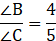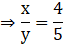⇒ 5x = 4y

From eq.

⇒ 5(90 - y) = 4y

⇒ 450 - 5y = 4y

⇒ 9y = 450

⇒ y = 50°

Putting this in eq.

⇒ x = 90 - 50 = 40°

Therefore, we have

∠A = x + y = 40° + 50° = 90°

∠B = x = 40°

∠C = y = 50°

Question 9.

Divide a rope of length 560 cm into 2 parts such that twice the length of the smaller part is equal to 1/3 of the larger part. Then find the length of the larger part.

Let the length of smaller part be 'x' cm and larger part be 'y' cm.

Length of rope = 560 cm

⇒ x + y = 560

⇒ y = 560 - x eq.

Also,

Twice the length of smaller part is equal toof the larger part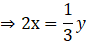⇒ 6x = y

⇒ 6x = 560 - x

⇒ 7x = 560

⇒ x = 80

Using this in eq.

⇒ y = 560 - 80 = 480

Therefore,

Length of smaller part = 'x' cm = 80 cm

Length of larger part = 'y' cm = 480 cm

Question 10.

In a competitive examination, there were 60 questions. The correct answer would carry 2 marks, and for incorrect answer 1 mark would be subtracted. Yashwant had attempted all the questions and he got total 90 marks. Then how many questions he got wrong?

Let the no of questions he got wrong be 'x'

And the no of questions he got right be 'y'

As, he attempted all the questions,

⇒ x + y = 60

⇒ y = 60 - x eq.

Also, he carries 2 for each corrects question and (-1) for each wrong question, also he got 90 marks

⇒ y(2) + x(-1) = 90

⇒ 2y - x = 90

⇒ 2(60 - x) - x = 90 eq.[Using 1]

⇒ 120 - 2x - x = 90

⇒ -3x = -30

⇒ x = 10

⇒ he got 10 wrong questions.

###### Problem Set 5
Question 1.

Choose the correct alternative answers for the following questions.

If 3x + 5y =9 and 5x + 3y =7 then What is the value of x + y?
A. 2

B. 16

C. 9

D. 7

3x + 5y = 9 eq.

5x + 3y = 7 eq.

Adding eq. and eq. we get

3x + 5y + 5x + 3y = 9 + 7

⇒ 8x + 8y = 16

Dividing both side by 8, we get

⇒ x + y = 2

Question 2.

'When 5 is subtracted from length and breadth of the rectangle, the perimeter becomes 26.' What is the mathematical form of the statement?
A. x – y = 8

B. x + y = 8

C. x + y =23

D. 2x + y = 21

Let the length be 'x' and breadth be 'y' units.

Perimeter of triangle = 2(x + y) units

If 5 is subtracted from length and breadth

Perimeter = 26 units eq.[Given]

⇒ 2( x - 5 + y - 5) = 26

⇒ 2(x + y - 10) = 26

⇒ x + y - 10 = 13

⇒ x + y = 23

Question 3.

Ajay is younger than Vijay by 5 years. Sum of their ages is 25 years. What is Ajay's age?
A. 20

B. 15

C. 10

D. 5

Let Ajay's age be 'x' years and Vijay's age be 'y' years.

Given, Ajay is younger than Vijay by 5 years

⇒ x = y - 5 eq.

Also, Sum of their ages is 25 years,

⇒ x + y = 25

From eq.

⇒ y - 5 + y = 25

⇒ 2y = 30

⇒ y = 15

Putting this in eq.

⇒ x = 15 - 5 = 10

Age of Ajay = x = 10 Years

Age of Vijay = y = 15 Years

Question 4.

Solve the following simultaneous equations.
i. 2x + y = 5; 3x - y = 5

ii. x - 2y = -1; 2x - y = 7

iii. x + y = 11; 2x - 3y = 7

iv. 2x + y = -2; 3x - y = 7

v. 2x - y = 5; 3x + 2y = 11

vi. x - 2y = -2; x + 2y = 10

(i)

2x + y = 5

⇒ y = 5 - 2x eq.

3x - y = 5

Using eq. we have

⇒ 3x - (5 - 2x) = 5

⇒ 3x - 5 + 2x = 5

⇒ 5x = 10

⇒ x = 2

Using 'x' in eq.

⇒ y = 5 - 2(2)

⇒ y = 5 - 4 = 1 cm

(ii)

x - 2y = -1

⇒ x = 2y - 1 eq.

2x - y = 7

Using eq., we have

⇒ 2(2y - 1) - y = 7

⇒ 4y - 2 - y = 7

⇒ 3y = 9

⇒ y = 3

Using this value in eq.

⇒ x = 2(3) - 1

⇒ x = 5

(iii)

x + y = 11

⇒ y = 11 - x eq.

2x - 3y = 7

Using eq., we have

⇒ 2x - 3(11 - x) = 7

⇒ 2x - 33 + 3x = 7

⇒ 5x = 40

⇒ x = 8

Using this in eq.

⇒ y = 11 - 8

⇒ y = 3

(iv)

2x + y = -2

⇒ y = -2x - 2 eq.

3x - y = 7

Using eq.

3x - (-2x - 2) = 7

⇒ 3x + 2x + 2 =7

⇒ 5x = 5

⇒ x = 1

Using this in eq.

⇒ y = -2(1) - 2

⇒ y = -2 - 2 = -4

(v)

2x - y = 5

⇒ y = 2x - 5 eq.

3x + 2y = 11

Using eq.

⇒ 3x + 2(2x - 5) = 11

⇒ 3x + 4x - 10 = 11

⇒ 7x = 21

⇒ x = 3

Using this in eq.

⇒ y = 2(3) - 5

⇒ y = 1

(vi)

x - 2y = -2

x = 2y - 2 eq.

x + 2y = 10

using eq., we have

⇒ 2y - 2 + 2y = 10

⇒ 4y = 12

⇒ y = 3

Using this in eq.

⇒ x = 2(3) - 2

⇒ x = 4

Question 5.

By equating coefficients of variables, solve the following equations.

i. 3x - 4y = 7; 5x + 2y = 3

ii. 5x + 7y = 17; 3x - 2y = 4

iii. x - 2y = -10; 3x - 5y = -12

iv. 4x + y = 34; x + 4y = 16

(i)

3x - 4y = 7 eq.

5x + 2y = 3 eq.

Multiplying eq. by 2 both side, we get

10x + 4y = 6 eq.

Adding eq. and eq., we get

3x - 4y + 10x + 4y = 7 + 6

⇒ 13x = 13

⇒ x = 1

Putting this in eq., we get

3(1) - 4y = 7

⇒ -4y = 7 - 3

⇒ -4y = 4

⇒ y = -1

(ii)

5x + 7y = 17 eq.

3x - 2y = 4 eq.

Multiplying eq. by 3 both side and Multiplying eq. by 5 both side we get,

15x + 21y = 51 eq.

15x - 10y = 20 eq.

Subtracting eq. from eq., we get

15x + 21y - 15x + 10y = 51 - 20

⇒ 31y = 31

⇒ y = 1

Putting this in eq., we get

5x + 7(1) = 17

⇒ 5x = 10

⇒ x = 2

(iii)

x - 2y = -10 eq.

3x - 5y = -12 eq.

Multiplying eq. by 3

3x - 6y = -30 eq.

Subtracting eq. from eq., we get

3x - 6y - 3x + 5y = -30 + 12

⇒ -y = -18

⇒ y = 18

Putting this in eq., we get

x - 2(18) = -10

⇒ x - 36 = -10

⇒ x = 26

(iv)

4x + y = 34 eq.

x + 4y = 16 eq.

Multiplying eq. by 4 both side, we get

4x + 16y = 64 eq.

Subtracting eq. from eq., we get

4x + 16y - 4x - y = 64 - 34

⇒ 15y = 30

⇒ y = 2

Putting this in eq., we get

x + 4(2) = 16

⇒ x + 8 = 16

⇒ x = 8

Question 6.

Solve the following simultaneous equations.
i.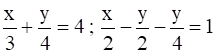ii.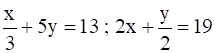iii.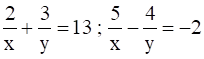(i)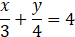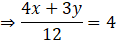⇒ 4x + 3y = 48 eq.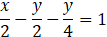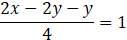⇒ 2x - 3y = 4 eq.

Adding eq. and eq., we get

⇒ 4x + 3y + 2x - 3y = 48 + 4

⇒ 6x = 52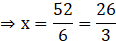Using this in eq., we have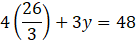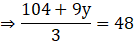⇒ 104 + 9y = 144

⇒ 9y = 40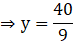(ii)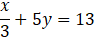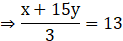⇒ x + 15y = 39

⇒ x = 39 - 15y eq.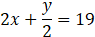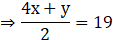⇒ 4x + y = 38

Using eq., we have

⇒ 4(39 - 15y) + y = 38

⇒ 156 - 60y + y = 38

⇒ 59y = 118

⇒ y = 2

Putting this value in eq.

⇒ x = 39 - 15(2)

⇒ x = 39 - 30

⇒ x = 9

(iii)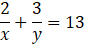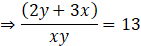⇒ 2y + 3x = 13xy eq.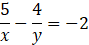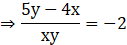⇒ 5y - 4x = -2xy eq.

Multiplying eq. by 4 both side, and Multiplying eq. by 3 both side, we get

8y + 12x = 52xy eq.

15y - 12x = -6xy eq.

⇒ 8y + 12x + 15y - 12x = 52xy - 6xy

⇒ 23y = 46xy

⇒ 1 = 2x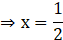Putting this in eq.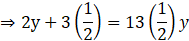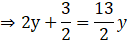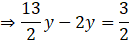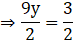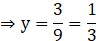Question 7.

A two-digit number is 3 more than 4 times the sum of its digits. If 18 is added to this number, the sum is equal to the number obtained by interchanging the digits. Find the number.

Let the unit digit be 'x'

Let the digit at ten's place be 'y'

The original number will be 10y + x

Given, number is 3 more than 4 times the sum of its digits

⇒ 10y + x = 4(x + y) + 3

⇒ 10y + x = 4x + 4y + 3

⇒ 6y - 3x = 3

⇒ 2y - x = 1

⇒ x = 2y - 1 eq.

Also,

If the digits are interchanged,

Reversed number will be = 10x + y

As, reversed number exceeds the original number by 18,

⇒ (10x + y) - (10y + x) = 18

⇒ 10x + y - 10y - x = 18

⇒ 9x - 9y = 18

⇒ x - y = 2

⇒ 2y - 1 - y = 2 eq.[using 1]

⇒ y = 3

Using this in eq.

⇒ x = 2(3) - 1 = 5

Hence the original number is 10y + x = 10(3) + 5 = 30 + 5 = 35.

Question 8.

The total cost of 6 books and 7 pens is 79 rupees and the total cost of 7 books and 5 pens is 77 ruppees. Find the cost of 1 book and 2 pens.

Let the cost of one book be 'x' rupees and cost of one pen be 'y' rupees.

Cost of 6 books and 7 pens = 79 Rs

⇒ 6x + 7y = 79 eq.

Cost of 7 books and 5 pens = 77 Rs

⇒ 7x + 5y = 77 eq.

Multiplying eq. by 5 both side, and Multiplying eq. by 7 both side, we get

⇒ 30x + 35y = 395 eq.

⇒ 49x + 35y = 539 eq.

Subtracting eq. from eq., we get

⇒ 49x + 35y - 30x - 35y = 539 - 395

⇒ 19x = 144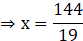Using this in eq.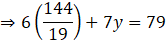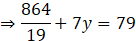⇒ 864 + 19×7y = 79×19

⇒ 19×7y = 79×19 – 864

⇒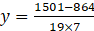⇒ y = 5

& 6x + 7y = 79

⇒ 6x + 35 = 79

⇒ 6x = 44

⇒ x = 7

Hence, the cost of 1 pen & 2 books = Rs 1(y) + 2x

= 5 + 14 = Rs 19.

Question 9.

The ratio of incomes of two persons is 9 : 7. The ratio of their expenses is 4 : 3. Every person saves rupees 200, find the income of each.

As the ratio of incomes is 9 : 7,

Let income of first person = 9x

Income of second person = 7x

Also, ratio of incomes is 4 : 3,

Let expenses of first person = 4y

Expenses of second person = 3y

Each person saves 200 Rs,

⇒ 9x - 4y = 200 eq.

⇒ 7x - 3y = 200 eq.

Multiplying eq. by 3 both side and Multiplying eq. by 4 both side, we get

⇒ 27x - 12y = 600 eq.

⇒ 28x - 12y = 800 eq.

Subtracting eq. from eq., we get

⇒ 28x - 12y - (27x - 12y) = 800 - 600

⇒ 28x - 12y - 27x + 12y = 200

⇒ x = 200

Income of first person = 9x = 9(200) = 1800 Rs

Income of second person = 7x = 7(200) = 1400 Rs

Question 10.

If the length of a rectangle is reduced by 5 units and its breadth is increased by 3 units, then the area of the rectangle is reduced by 8 square units. If length is reduced by 3 units and breadth is increased by 2 units, then the area of rectangle will increase by 67 square units. Then find the length and breadth of the rectangle.

Let the length be 'x' and breadth be 'y'

Area of rectangle = length × breadth

Area of rectangle = xy

First case:

Length = x - 5

As, area is reduced by 8 sq. units

⇒ xy - (x - 5)(y + 3) = 8

⇒ xy - (xy + 3x - 5y - 15) = 8

⇒ xy - xy - 3x + 5y + 15 = 8

⇒ 3x - 5y = 7 eq.

Second case:

Length = x - 3

As, the area is increased by 67 units

⇒ (x - 3)(y + 2) - xy = 67

⇒ xy + 2x - 3y - 6 - xy = 67

⇒ 2x - 3y = 73 eq.

Multiplying eq. by 2 both side, and Multiplying eq. by 3 both side, we get

⇒ 6x - 10y = 14 eq.

⇒ 6x - 9y = 219 eq.

Subtracting eq. from eq.

⇒ 6x - 9y - 6x + 10y = 219 - 14

⇒ y = 205

Using this in eq.

⇒ 3x - 5(205) = 7

⇒ 3x - 1025 = 7

⇒ 3x = 1032

⇒ x = 344

Hence, length = x = 344 units

Breadth = y = 219 units.

Question 11.

The distance between two places A and B on road is 70 kilometers. A car starts from A and the other from B. If they travel in the same direction, they will meet after 7 hours. If they travel towards each other they will meet after 1 hour, then find their speeds.

Let the speed of car at place A is x km/h and that of car at place B is y km/h

If they travel in same direction, they will meet after 7 hours, i.e. the difference of distance covered by them in 7 hours will be equal to distance b/w A and B.

As, distance = speed × time, and distance from A to B is 70 km

⇒ 7x - 7y = 70

⇒ x - y = 10

⇒ x = y + 10 eq.

If they, travel in opposite direction, they will meet after 1 hour i.e. sum of distance travelled by both cars will be equal to the distance b/w A and B.

⇒ x + y = 70

Using eq., we have

⇒ y + 10 + y = 70

⇒ 2y = 60

⇒ y = 30

Using this in eq., we have

x = 30 + 10 = 40

Hence,

Speed of car at A = x = 40 km/h

Speed of car at B = y = 30 km/h

Question 12.

The sum of a two-digit number and the number obtained by interchanging its digits is 99. Find the number.

Let the unit digit be 'x' and digit at ten's place be 'y'

Original Number = 10y + x

Number obtained by interchanging digits = 10x + y

Given,

10y + x + 10x + y = 99

⇒ 11x + 11y = 99

⇒ x + y = 9

If x = 1, y = 8 and number is 18

If x = 2, y = 7 and number is 27

If x = 3, y = 6 and number is 36

If x = 4, y = 5 and number is 45

If x = 5, y = 4 and number is 54

If x = 6, y = 3 and number is 63

If x = 7, y = 2 and number is 72

If x = 8, y = 1 and number is 81